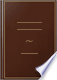Inicia la sessió
 Llibres Llibres 41 - 50 de 195 sobre Parallelograms upon the same base and between the same parallels, are equal to one....Parallelograms upon the same base and between the same parallels, are equal to one another.Elements of Geometry: Containing the First Six Books of Euclid, with a ... - Pàgina 41
per Euclid, John Playfair - 1826 - 320 pàgines
Visualització completa - Sobre aquest llibre## The works of William Cowper, with a life of the author, by the editor R. Southey

Robert Southey - 1843
...angles, or are together equal to two right angles. 2. Define a parallelogram. Parallelograms on the same base and between the same parallels are equal to one another. Shew that if any quadrilateral figure be bisected by both its diagonals it is a parallelogram. 3. To...
Visualització completa - Sobre aquest llibre## The British Annals of Education for ...: Being The Scholastic ..., Volum 1

1844
...MODERATOR AND SENIOR EXAMINER. [The Differential Calculut it not to be wed.] 1 . Parallelograms on the same base and between the same parallels are equal to one another. 2. From a given circle to cut off a segment containing an angle equal to a given rectilineal angle....
Visualització completa - Sobre aquest llibre## Euclid's Elements of geometry [book 1-6, 11,12] with explanatory notes ...

Robert Potts - 1845
...divides the parallelogram ACDB into two equal parts. QED PROPOSITION XXXV. THEOREM. Parallelograms upon the same base, and between the same parallels, are equal to one another. Let the parallelograms ABCD, EBCF be upon the same base BC, and between the same parallels AF, BC. Then the...
Visualització completa - Sobre aquest llibre## Euclid in Paragraphs: The Elements of Euclid: Containing the First Six Books ...

Euclid - 1845 - 199 pàgines
...ACDB into two equal parts. QED BOOK I. PROP. XXXV. XXXVI. PROPOSITION XXXV. THEOR. — Parallelograms upon the same base, and between the same parallels, are equal to one another. Let the parallelograms ABCD, EBCF be upon the same See the 2nd base BC, and between the same parallels AF,...
Visualització completa - Sobre aquest llibre## The first six and the eleventh and twelfth books of Euclid's Elements; with ...

James Thomson - 1845 - 358 pàgines
...parallelogram AC is equal (I. ax. 1) to EG. Wherefore parallelograms, &c. PROP. XXXVII. TnEOR.t — Triangles upon the same base, and between the same parallels, are equal to one another. * lt will appear, from this proposition, that the perimeters of two equal parallelograms on the same...
Visualització completa - Sobre aquest llibre## A Course of Practical Geometry for mechanics

William Pease - 1846
...form the isosceles triangle required. The reason of this is (Prob. XXXVII. Bk. I. Euclid,) because triangles upon the same base, and between the same parallels, are equal to one another : ie the triangles ACB and AE B, being upon the base, AB, to which the line EC is parallel, therefore...
Visualització completa - Sobre aquest llibre## The Elements of Geometry, Symbolically Arranged

1846
...•=• parts. Wherefore the opposite sides and angles, &c. PROP. XXXIV. THEOR. 3s. lEu. Parallelograms upon the same base, and between the same parallels, are equal to one another. PROP. XXXIV. FADE F \ . If the sides AD, DF, of the 1=1™ ABCD, DBCF, opp. to BC the base, be terminated...
Visualització completa - Sobre aquest llibre## The Elements of Euclid, the parts read in the University of Cambridge [book ...

Euclides - 1846
...to the triangle DBC. Wherefore, Triangles %c. QED PROP. XXXVIII. THEOR. Triangles upon equal bases, and between the same parallels, are equal to one another. Let the triangles ABC, DEF be upon equal bases BC, EF, and between the same parallels BF, AD : the triangle ABC shall be equal...
Visualització completa - Sobre aquest llibre## The Elements of Geometry, Symbolically Arranged

1846
...two = parts. Wherefore the opposite sides and angles, &c. PROP. XXXIV. THEOR. 35. lEu. Parallelograms upon the same base, and be-tween the same parallels, are equal to one another. FA DEFAEDF \ . If the sides AD, DF, of the / — 7"" ABCD, DBCF, opp. to RC the base, be terminated...
Visualització completa - Sobre aquest llibre## THE LONDON UNIVERSITY CALENDAR

...exterior angles of any rectilineal figure are together equal to four right angles. 6. Parallelograms upon the same base and between the same parallels are equal to one another. 7. Show that the complements of the parallelograms which are about the diameter of any .parallelogram...
Visualització completa - Sobre aquest llibre